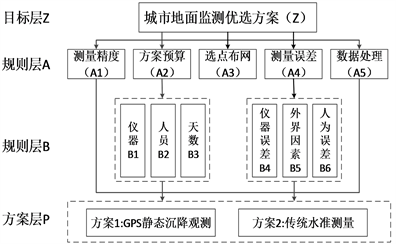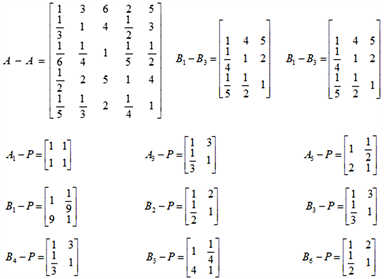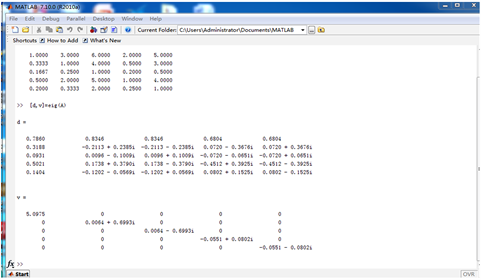# 基于层次分析法的城市沉降监测方案优选An Optimal Selection Method of Urban Subsidence Monitoring Based on AHP

DOI: 10.12677/GSER.2021.101005, PDF, HTML, XML, 下载: 49  浏览: 94

Abstract: Subsidence monitoring can effectively reflect the changes of ground elevation in time and provide important technical support and decision-making suggestions for the rapid development of cities. However, the selection of urban subsidence monitoring is often affected by factors such as budget, region, etc., the traditional method is arbitrary and subjective, and difficult to balance the observa-tion accuracy and economic cost. To solve the above problems, this paper proposes an optimal se-lection method of urban subsidence monitoring based on AHP. Through the construction of judg-ment matrix, consistency test and weight calculation of influence factors, the qualitative and quan-titative decision analysis method is used to comprehensively evaluate the land subsidence observa-tion scheme in a specific city. The experimental results show that the scheme of using GPS to moni-tor the land subsidence in this city is better than the traditional leveling. The GPS observation can meet the accuracy and effectively save manpower, time and economic costs. The relevant results can provide reference for the selection of other surveying and mapping projects.

1. 引言

2. 方法

2.1. 城市地面沉降监测层次结构模型Figure 1. Urban settlement monitoring scheme structure hierarchy diagram

2.2. 基于专家经验构建判断矩阵

$A=\left[\begin{array}{cc}\begin{array}{cc}{a}_{11}& {a}_{12}\\ {a}_{21}& {a}_{22}\end{array}& \begin{array}{cc}\cdots & {a}_{1n}\\ \cdots & {a}_{2n}\end{array}\\ \begin{array}{cc}⋮& ⋮\\ {a}_{n1}& {a}_{n1}\end{array}& \begin{array}{cc}\ddots & ⋮\\ \cdots & {a}_{nn}\end{array}\end{array}\right]$

2.3. 层次单排序及一致性检验

${a}_{ij}=\frac{{a}_{ij}}{{\sum }_{i=1}^{n}{a}_{ij}}$ (1)

${w}_{i}={\sum }_{j=1}^{n}{a}_{ij}$ (2)

${w}_{i}=\frac{{w}_{i}}{{\sum }_{j=1}^{n}{w}_{i}}$ (3)

${\lambda }_{\mathrm{max}}={\sum }_{i=1}^{n}\frac{{\left[Aw\right]}_{i}}{n{w}_{i}}$ (4)

$CI=\frac{{\lambda }_{\mathrm{max}}-n}{n-1}$ (5)

$CR=\frac{CI}{RI}$ (6)

2.4. 层次总排序及一致性检验

${P}_{i}={\sum }_{j=1}^{m}{a}_{j}{b}_{ij}$ (7)

$\begin{array}{l}{B}_{1}:{a}_{1}{b}_{11}+{a}_{2}{b}_{12}+\cdots +{a}_{m}{b}_{1m}\\ {B}_{2}:{a}_{1}{b}_{21}+{a}_{2}{b}_{22}+\cdots +{a}_{m}{b}_{2m}\\ \text{\hspace{0.17em}}\text{\hspace{0.17em}}\text{\hspace{0.17em}}\text{\hspace{0.17em}}\text{\hspace{0.17em}}\text{\hspace{0.17em}}\text{\hspace{0.17em}}\text{\hspace{0.17em}}\text{\hspace{0.17em}}\text{\hspace{0.17em}}\text{\hspace{0.17em}}\text{\hspace{0.17em}}\text{\hspace{0.17em}}\text{\hspace{0.17em}}\text{\hspace{0.17em}}\text{\hspace{0.17em}}⋮\\ {B}_{n}:{a}_{1}{b}_{n1}+{a}_{2}{b}_{n2}+\cdots +{a}_{m}{b}_{nm}\end{array}$ (8)

$CR=\frac{{a}_{1}C{I}_{1}+{a}_{2}C{I}_{2}+\cdots +{a}_{m}C{I}_{m}}{{a}_{1}R{I}_{1}+{a}_{2}R{I}_{2}+\cdots +{a}_{m}R{I}_{m}}$ (9)

3. 案例分析Figure 2. The monitoring scheme selects the judgment matrix of each levelFigure 3. Matlab calculation results

4. 结束语

  王建文. 精密水准测量技术在重点沉降区监测中的应用[D]: [硕士学位论文]. 西安: 西安科技大学, 2020.  兰徽. 全站仪水准法在基坑沉降监测中的分析与应用[J]. 福建建材, 2020(8): 20-21+27.  谢旭晖, 许锡文. 基于高精度水准测量的高层建筑沉降观测[J]. 江西建材, 2018(13): 41-42.  王雷霆. 论精密水准测量在地面沉降监测中的应用[J]. 华北国土资源, 2013(2): 113-116.  秦洪奎, 王平德. GPS用于天津市地面沉降监测的研究[J]. 测绘信息与工程, 2012, 37(2): 20-21.  徐自平. 数字水准仪在沉降观测中的应用[J]. 山西建筑, 2019, 45(22): 146-148.  林立祥. 全站仪三角高程法在路面沉降观测中的应用[J]. 土工基础, 2019, 33(2): 215-219.  王明月, 潘颖, 花阳. 大型商业建筑沉降观测方法浅析[J]. 现代测绘, 2015, 38(2): 23-25.  Chen, J., Yue, D., Liu, Z., et al. (2019) Experimental Research on Daily Deformation Monitoring of Bridge Using BDS/GPS. Survey Review, 51, 472-482. https://doi.org/10.1080/00396265.2018.1481699  Tu, R., Liu, J., Lu, C., et al. (2017) Cooperating the BDS, GPS, GLONASS and Strong-Motion Observations for Real-Time Deformation Monitoring. Geophysical Journal International, 209, 1408-1417. https://doi.org/10.1093/gji/ggx099  胡丛玮, 刘大杰. 基于GPS基准站网的GPS测量[J]. 现代测绘, 2003(1): 10-13.  易长荣, 王淼, 徐冬. GPS连续站监测天津市地面沉降的初步结果[J]. 现代测绘, 2009, 32(1): 31-33.  朱庆, 陈凯峥, 谢潇, 等. 模糊层次分析与三维GIS集成的升压站选址方法[J]. 西南交通大学学报, 2019, 54(5): 980-988.  徐勇炜. 基于层次分析法的南陵县乡村旅游资源评价[J]. 安徽农学通报, 2020, 26(24): 159-162.  程寅, 陆凤, 范睿, 朱敏. 基于AHP构建城市生长地理适宜性表面模型研究[J]. 现代测绘, 2018, 41(6): 29-32.  贾涛. 成兰铁路松潘段泥石流灾害风险评估[D]: [硕士学位论文]. 成都: 成都理工大学, 2015.  刘厚成, 谷秀芝. 基于可拓层次分析法的泥石流危险性评价研究[J]. 中国地质灾害与防治学报, 2010, 21(3): 61-66.